# Basic Geometry : How to find the perimeter of a square

## Example Questions

← Previous 1 3 4 5 6 7 8

### Example Question #1 : How To Find The Perimeter Of A Square

The length of one side of a square is 6 inches.  What is the perimeter of the square?Explanation:

To find the perimeter of a square, multiply one of its sides by 4.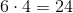### Example Question #2 : How To Find The Perimeter Of A Square

The area of squareis 196 square centimeters. What is the perimeter of?

Cannot be determined.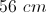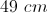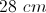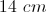Explanation:

We know that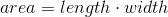.

We also know that sinceis a square, all of its sides are of equal length.

To get the measure of any one side of, take the square root of the area: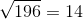centimeters

Therefore each side ofis 14 centimeters long.

To find the perimeter, multiply by 4: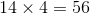centimeters

### Example Question #1 : How To Find The Perimeter Of A Square

A square tabletop has an area of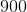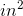. Find the perimeter of the tabletop.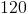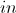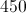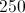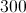Explanation:

First find the length of each side.

Take the square root of the area to find the side length: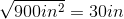Since a square hasequal sides, multiply the side length byto find the perimeter: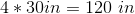### Example Question #4 : How To Find The Perimeter Of A Square

A square has an area of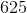, what is the perimeter?Explanation:

The area of a square is: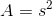, where s is the side length of the square.

So using the numbers: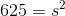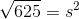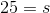Therefore, every side of the square is 25.

Now to find the perimeter, a square has 4 equal sides: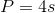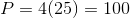The perimeter of the square is 100

### Example Question #1 : How To Find The Perimeter Of A Square

A square garden has an area of 64 square feet. If you add 3 feet to each side, what is the new perimeter of the garden?

32

25

20

44

121

44

Explanation:

By finding the square root of the area of the garden, you find the length of one side, which is 8. We add 3 feet to this, giving us 11, then multiply this by 4 to get 44 feet for the perimeter.

### Example Question #1 : How To Find The Perimeter Of A Square

The area of the shaded region of a square is 18. What is the perimeter of the square?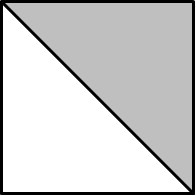24

36

28

20

24

Explanation:

The area of the shaded region, which covers half of the square is 18 meaning that the total area of the square is 18 x 2, or 36. The area of a square is equal to the length of one side squared. Since the square root of 36 is 6, the length of 1 side is 6. The perimeter is the length of 1 side times 4 or 6 x 4.

### Example Question #2 : How To Find The Perimeter Of A Square

The area of a square is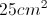.  If the square is enlarged by a factor of 2, what is the perimeter of the new square?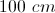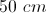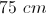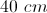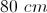Explanation:

The area of a square is given by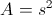so we know the side is 5 cm.  Enlarging by a factor of two makes the new side 10 cm.  The perimeter is given by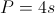, so the perimeter of the new square is 40 cm.

### Example Question #1 : How To Find The Perimeter Of A Square

The diagonal of a square has a length of 10 inches. What is the perimeter of the square in inches squared?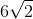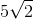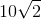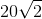Explanation:

Using the Pythagorean Theorem, we can find the edge of a side to be √50, by 2a2=102. This can be reduced to 5√2. This can then be multiplied by 4 to find the perimeter.

### Example Question #1 : How To Find The Perimeter Of A Square

What is the perimeter of a square with an area of?Explanation:

1. Find the side lengths: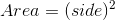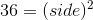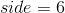2. Use the side lengths to find the perimeter: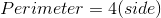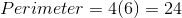### Example Question #10 : How To Find The Perimeter Of A Square

Find the perimeter of a square that has side lengths of.Explanation:

Use the following formula to find the perimeter of a square: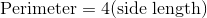For the given square,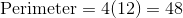← Previous 1 3 4 5 6 7 8

### All Basic Geometry Resources